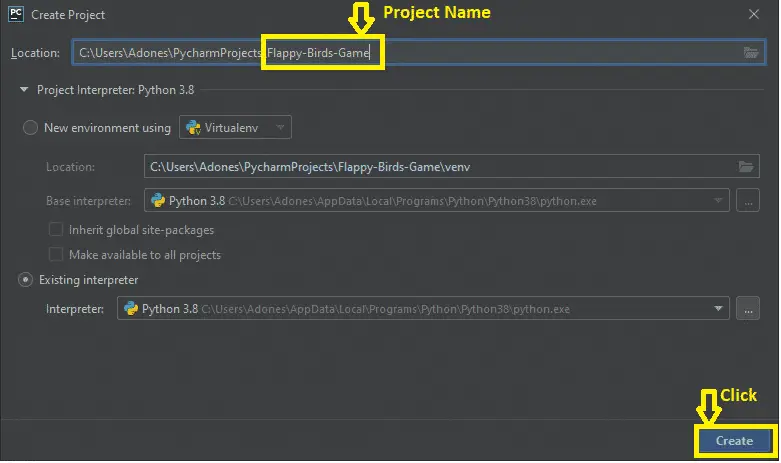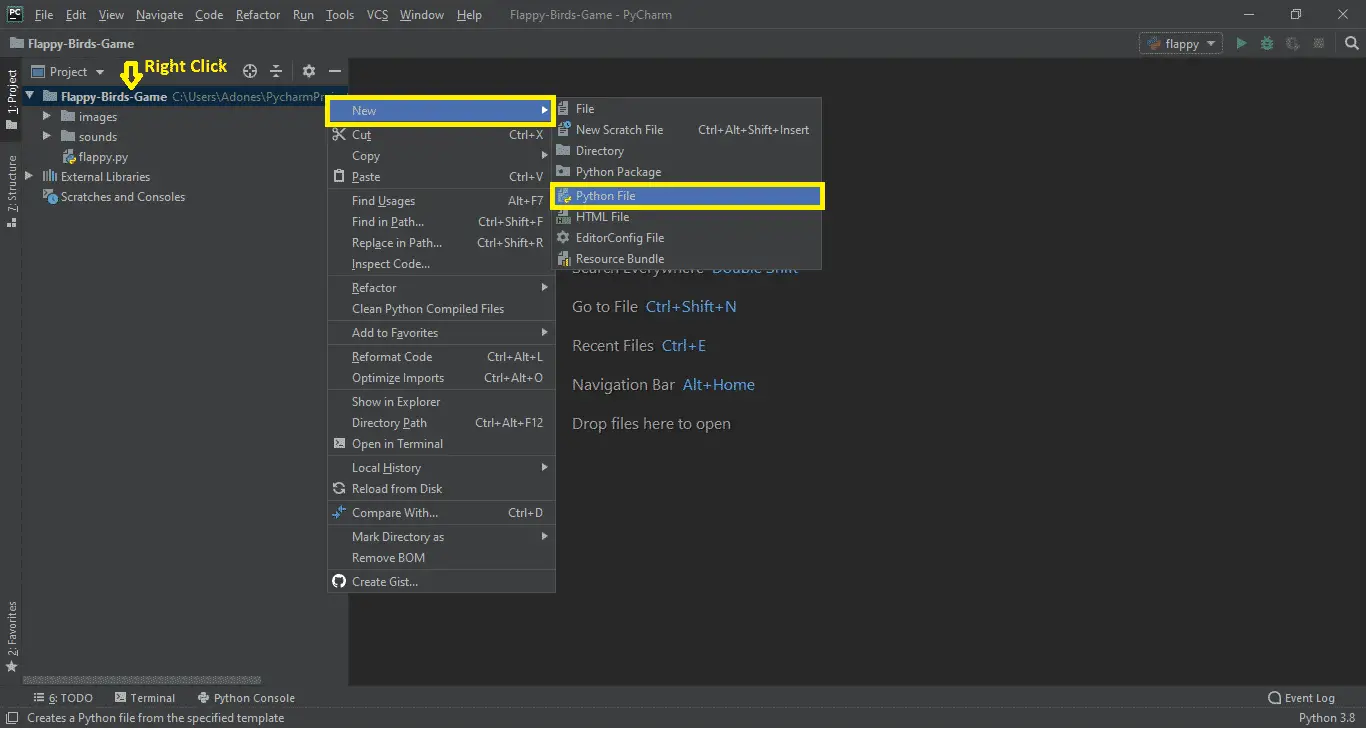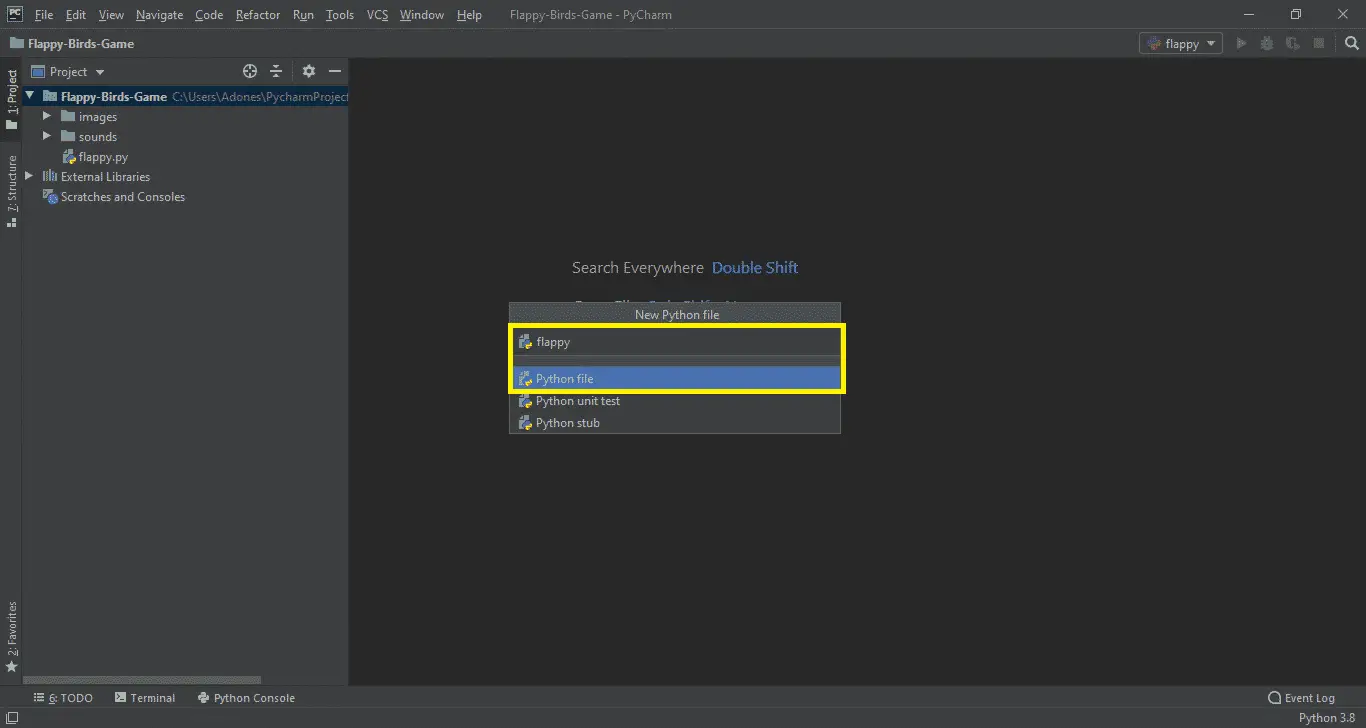# Flappy Bird Game in Python with Source Code

A Flappy Bird Game in Python is free to download the open source code and it is created for beginners who want to learn Python.

The project system used a Pygame and Random module. Pygame is a cross-platform set of Python modules designed for writing video games.

## Flappy Bird Code in Python – Project Information

This Flappy Bird Code has a task record that contains an image file, a sounds file, and a Python contents file (flappy.py).

The ongoing interaction Graphics is sufficient and the controls are straightforward for the clients.

Discussing the ongoing interaction, it’s one of the most addictive and messed around for all. All the playing strategies are too straightforward simply like the genuine one.

You should simply attempt to remain on the screen until long green channels show up before you.

Here, the client needs to control the winged animal fluttering up, and down utilizing Spacebar, without contacting pipes so as to score game focuses.

This implies the more you go through green funnels, the more will be the game focuses. A straightforward GUI has accommodated the simple ongoing interaction.

The ongoing interaction configuration is easy to such an extent that the client won’t think that it’s hard to utilize and explore.

Before you start on how to create Flappy Bird Game in Python, make sure that you have PyCharm IDE and Pygame installed on your computer.

## How to Create Flappy Bird in Python?

Time needed: 5 minutes

These are the steps on How to Create Flappy Bird in Python with Source Code

• Step 1: Create a project name.

First, when you finished installing the Pycharm IDE on your computer, open it and then create a “project name.” After creating a project name click the “create” button.• Step 2: Create a python file.

Second, after creating a project name, “right-click” your project name and then click “new.” After that click the “python file“.• Step 3: Name your python file.

Third, after creating a Python file, Name your Python file after that click “enter• Step 4: The actual code.

This is the actual coding on how to create Flappy Bird Game in Python, and you are free to copy this code and download the full source code given below.

## Code Explanations

### 1. Importing Random Module

Code:

```import random
```

Explanation:

In the code given below. which is for the random() function, which generates random numbers between 0 and 1.

### 2. Importing Pygame Module

Code:

```import pygame
from pygame.locals import *```

Explanation:

The code given below, which is pygame library is an open-source module for the Python programming language specifically intended to help you make games and other multimedia applications. Pygame can run across many platforms and operating systems.

### 3. Importing Sys Module

Code:

`import sys`

Explanation:

In the code given below, which is for the function of the sys module. We will use sys.exit to exit the program.

### 4. This module is for the global variables

Code:

```FPS = 32
scr_width = 289
scr_height = 511
display_screen_window = pygame.display.set_mode((scr_width, scr_height))
play_ground = scr_height * 0.8
game_image = {}
game_audio_sound = {}
player = 'images/bird.png'
bcg_image = 'images/background.png'
pipe_image = 'images/pipe.png'```

Explanation:

In the code given below, which is for the global variables for the game.

### 5. This module is for the welcome main screen

Code:

```def welcome_main_screen():
"""
Shows welcome images on the screen
"""

p_x = int(scr_width / 5)
p_y = int((scr_height - game_image['player'].get_height()) / 2)
msgx = int((scr_width - game_image['message'].get_width()) / 2)
msgy = int(scr_height * 0.13)
b_x = 0
while True:
for event in pygame.event.get():
# if user clicks on cross button, close the game
if event.type == QUIT or (event.type == KEYDOWN and event.key == K_ESCAPE):
pygame.quit()
sys.exit()

# If the user presses space or up key, start the game for them
elif event.type == KEYDOWN and (event.key == K_SPACE or event.key == K_UP):
return
else:
display_screen_window.blit(game_image['background'], (0, 0))
display_screen_window.blit(game_image['player'], (p_x, p_y))
display_screen_window.blit(game_image['message'], (msgx, msgy))
display_screen_window.blit(game_image['base'], (b_x, play_ground))
pygame.display.update()
time_clock.tick(FPS)```

Explanation:

In the code given below, which is for the function of the welcome main screen. It shows welcome images on the screen.

### 6. This module is for the main gameplay

Code:

```def main_gameplay():
score = 0
p_x = int(scr_width / 5)
p_y = int(scr_width / 2)
b_x = 0

n_pip1 = get_Random_Pipes()
n_pip2 = get_Random_Pipes()

up_pips = [
{'x': scr_width + 200, 'y': n_pip1['y']},
{'x': scr_width + 200 + (scr_width / 2), 'y': n_pip2['y']},
]

low_pips = [
{'x': scr_width + 200, 'y': n_pip1['y']},
{'x': scr_width + 200 + (scr_width / 2), 'y': n_pip2['y']},
]

pip_Vx = -4

p_vx = -9
p_mvx = 10
p_mvy = -8
p_accuracy = 1

p_flap_accuracy = -8
p_flap = False

while True:
for event in pygame.event.get():
if event.type == QUIT or (event.type == KEYDOWN and event.key == K_ESCAPE):
pygame.quit()
sys.exit()
if event.type == KEYDOWN and (event.key == K_SPACE or event.key == K_UP):
if p_y > 0:
p_vx = p_flap_accuracy
p_flap = True
game_audio_sound['wing'].play()

cr_tst = is_Colliding(p_x, p_y, up_pips,
low_pips)
if cr_tst:
return

p_middle_positions = p_x + game_image['player'].get_width() / 2
for pipe in up_pips:
pip_middle_positions = pipe['x'] + game_image['pipe'].get_width() / 2
if pip_middle_positions <= p_middle_positions < pip_middle_positions + 4:
score += 1
game_audio_sound['point'].play()

if p_vx < p_mvx and not p_flap:
p_vx += p_accuracy

if p_flap:
p_flap = False
p_height = game_image['player'].get_height()
p_y = p_y + min(p_vx, play_ground - p_y - p_height)

for pip_upper, pip_lower in zip(up_pips, low_pips):
pip_upper['x'] += pip_Vx
pip_lower['x'] += pip_Vx

if 0 < up_pips['x'] < 5:
new_pip = get_Random_Pipes()
up_pips.append(new_pip)
low_pips.append(new_pip)

if up_pips['x'] < -game_image['pipe'].get_width():
up_pips.pop(0)
low_pips.pop(0)

display_screen_window.blit(game_image['background'], (0, 0))
for pip_upper, pip_lower in zip(up_pips, low_pips):
display_screen_window.blit(game_image['pipe'], (pip_upper['x'], pip_upper['y']))
display_screen_window.blit(game_image['pipe'], (pip_lower['x'], pip_lower['y']))

display_screen_window.blit(game_image['base'], (b_x, play_ground))
display_screen_window.blit(game_image['player'], (p_x, p_y))
d = [int(x) for x in list(str(score))]
w = 0
for digit in d:
w += game_image['numbers'][digit].get_width()
Xoffset = (scr_width - w) / 2

for digit in d:
display_screen_window.blit(game_image['numbers'][digit], (Xoffset, scr_height * 0.12))
Xoffset += game_image['numbers'][digit].get_width()
pygame.display.update()
time_clock.tick(FPS)
```

Explanation:

The code is given below, which is for the function of the main gameplay of a game. Create 2 pipes for blitting on the screen. Includes the list of upper and lower pipes. Checking for the score function, Add a new pipe when the first is about to cross the leftmost part of the screen.

### 7. This module is for the get random pipes

Code:

```def get_Random_Pipes():
pip_h = game_image['pipe'].get_height()
off_s = scr_height / 3
yes2 = off_s + random.randrange(0, int(scr_height - game_image['base'].get_height() - 1.2 * off_s))
pipeX = scr_width + 10
y1 = pip_h - yes2 + off_s
pipe = [
{'x': pipeX, 'y': -y1}, # upper Pipe
{'x': pipeX, 'y': yes2} # lower Pipe
]
return pipe```

Explanation:

In the code given below, which is for the function of getting random pipes. Generate positions of two pipes(one bottom straight and one top rotated ) for blitting on the screen.

### 8. This module is for images

Code:

```if __name__ == "__main__":

pygame.init()
time_clock = pygame.time.Clock()
pygame.display.set_caption('Flappy Bird Game')
game_image['numbers'] = (
)

)```

Explanation:

The code given below is for the function of images used in a game.

### 9. This module is for the sound

Code:

```game_audio_sound['die'] = pygame.mixer.Sound('sounds/die.wav')
game_audio_sound['hit'] = pygame.mixer.Sound('sounds/hit.wav')
game_audio_sound['point'] = pygame.mixer.Sound('sounds/point.wav')
game_audio_sound['swoosh'] = pygame.mixer.Sound('sounds/swoosh.wav')
game_audio_sound['wing'] = pygame.mixer.Sound('sounds/wing.wav')
```

Explanation:

The code is given below, which is for the function of sound/audio use in a game.

## Summary

That’s how you create a Flappy Bird Game in Python in your projects. You can always expand and try different ways of implementing the Flappy Bird Code in your Python projects.

Flappy Bird Python is free to download the open source code and it is used for educational purposes only.

## Inquiries

### 3 thoughts on “Flappy Bird Game in Python with Source Code”

1.yeeeeeeeeeeeeeeeeeeeeeeeeeeeeeeeeeeeeeeeeeeeeeeeeeeee

2.Wow this post changed my life for the better. Thank you so very ungodly much

3.What module is for U-R: A.S.S.?